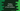# Python program to find the gcd of two numbers using fractions module## Introduction :

Python fractions module provides support for rational number arithmetic. It comes with one method for calculating gcd of two numbers easily. In this tutorial, we will learn how to calculate the gcd of two numbers using fractions module. The user will input both numbers and the program will calculate and print the gcd of the numbers.

## Definition of fractions.gcd :

The gcd function is defined as below :

``fractions.gcd(a, b)``

This method takes two integers as arguments and returns the greatest common divisor of these numbers. The gcd is the largest integer that divides both a and b if either a or b is nonzero. If both are 0, it will return 0. If b is nonzero, gcd will take the sign of b, else it will have the same sign as a.

## Python program :

``````//1
import fractions

//2
num1 = int(input("Enter the first number : "))
num2 = int(input("Enter the second number : "))

//3
print("The gcd of {} and {} is {}".format(num1, num2, fractions.gcd(num1, num2)))``````

You can download this program from here.

### Explanation :

The commented numbers in the above program denote the step numbers below :

1. Import fractions module.
2. Ask the user to enter the first and second number. Read the numbers and store them in num1 and num2 variables.
3. Print the gcd of both numbers using _fractions.gcd _method.

### Sample Output :

``````Enter the first number : 12
Enter the second number : 24
The gcd of 12 and 24 is 12

Enter the first number : 2004
Enter the second number : 100
The gcd of 2004 and 100 is 4

Enter the first number : 0
Enter the second number : 0
The gcd of 0 and 0 is 0``````